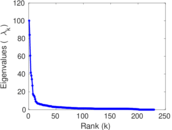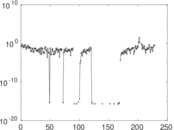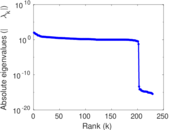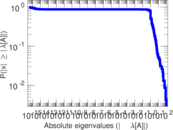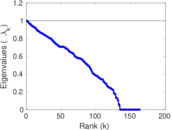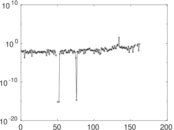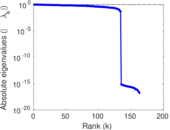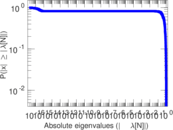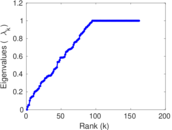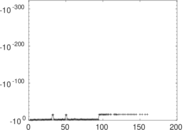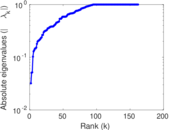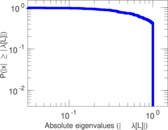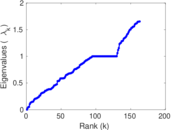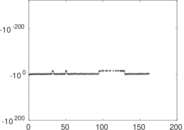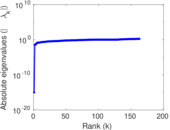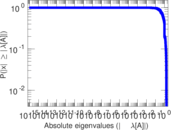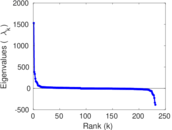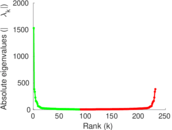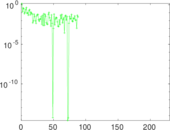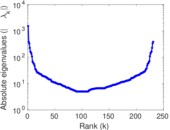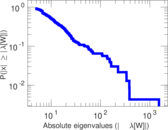# Wiktionary edits (ss)

This is the bipartite edit network of the Swati Wiktionary. It contains users and pages from the Swati Wiktionary, connected by edit events. Each edge represents an edit. The dataset includes the timestamp of each edit.

 Code `mss` Internal name `edit-sswiktionary` Name Wiktionary edits (ss) Data source http://dumps.wikimedia.org/ AvailabilityDataset is available for download Consistency checkDataset passed all tests Category Authorship network Dataset timestamp 2017-10-20 Node meaning User, article Edge meaning Edit Network formatBipartite, undirected Edge typeUnweighted, multiple edges Temporal dataEdges are annotated with timestamps

## Statistics

 Size n = 1,540 Left size n1 = 231 Right size n2 = 1,309 Volume m = 4,703 Unique edge count m̿ = 2,776 Wedge count s = 329,959 Claw count z = 44,574,233 Cross count x = 5,271,041,510 Square count q = 127,193 4-Tour count T4 = 2,345,436 Maximum degree dmax = 1,050 Maximum left degree d1max = 1,050 Maximum right degree d2max = 145 Average degree d = 6.107 79 Average left degree d1 = 20.359 3 Average right degree d2 = 3.592 82 Fill p = 0.009 180 53 Average edge multiplicity m̃ = 1.694 16 Size of LCC N = 1,339 Diameter δ = 11 50-Percentile effective diameter δ0.5 = 3.417 73 90-Percentile effective diameter δ0.9 = 5.656 30 Median distance δM = 4 Mean distance δm = 3.933 98 Gini coefficient G = 0.732 677 Balanced inequality ratio P = 0.213 906 Left balanced inequality ratio P1 = 0.108 654 Right balanced inequality ratio P2 = 0.289 815 Relative edge distribution entropy Her = 0.792 558 Power law exponent γ = 2.741 89 Tail power law exponent γt = 2.361 00 Tail power law exponent with p γ3 = 2.361 00 p-value p = 0.000 00 Left tail power law exponent with p γ3,1 = 1.791 00 Left p-value p1 = 0.780 000 Right tail power law exponent with p γ3,2 = 8.281 00 Right p-value p2 = 0.677 000 Degree assortativity ρ = −0.193 659 Degree assortativity p-value pρ = 7.307 35 × 10−25 Spectral norm α = 100.094 Algebraic connectivity a = 0.031 991 9 Spectral separation |λ1[A] / λ2[A]| = 1.190 28 Controllability C = 1,108 Relative controllability Cr = 0.729 908

## Plots

### Fruchterman–Reingold graph drawing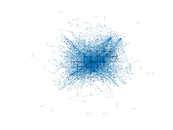### Degree distribution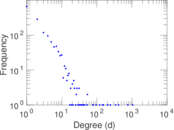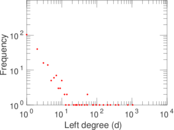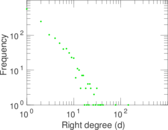### Cumulative degree distribution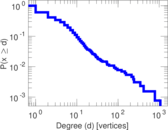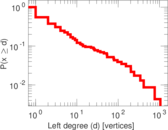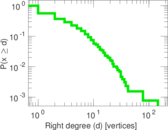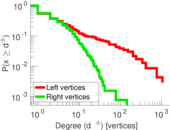### Lorenz curve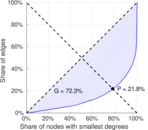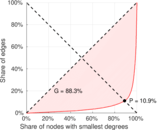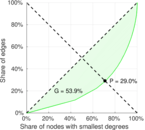### Spectral distribution of the adjacency matrix### Spectral distribution of the normalized adjacency matrix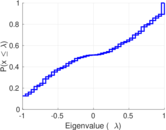### Spectral distribution of the Laplacian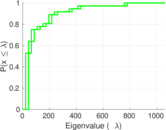### Spectral graph drawing based on the adjacency matrix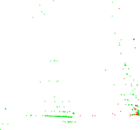### Spectral graph drawing based on the Laplacian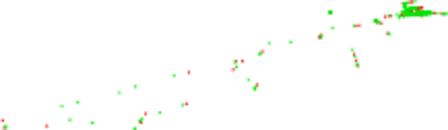### Spectral graph drawing based on the normalized adjacency matrix### Degree assortativity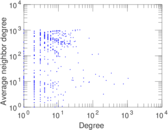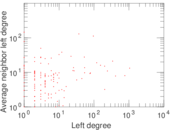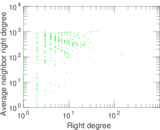### Zipf plot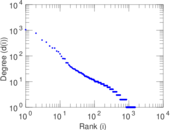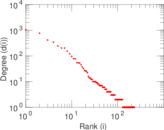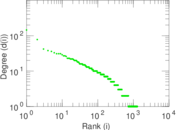### Hop distribution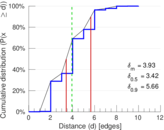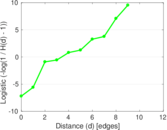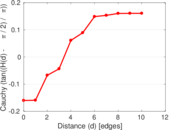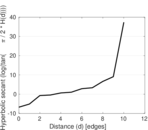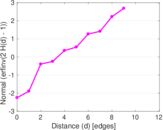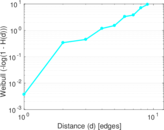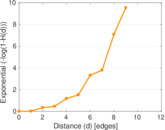### Double Laplacian graph drawing### Delaunay graph drawing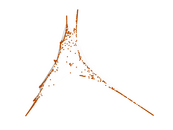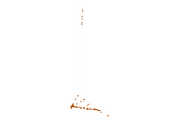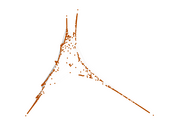### Edge weight/multiplicity distribution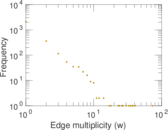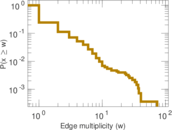### Temporal distribution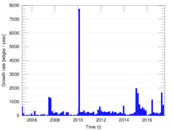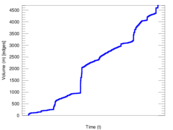### Temporal hop distribution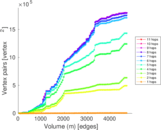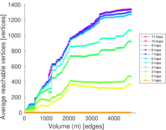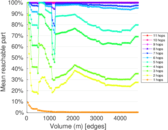### Diameter/density evolution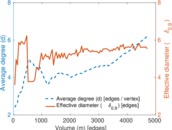### Matrix decompositions plots